# 为什么0.1 + 0.2不等于0.3？

0.1 + 0.2不等于0.3这是一个普遍的问题，例如在JS控制台输入将得到0.30000000000000004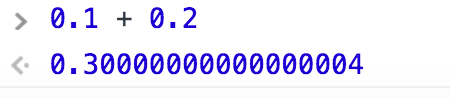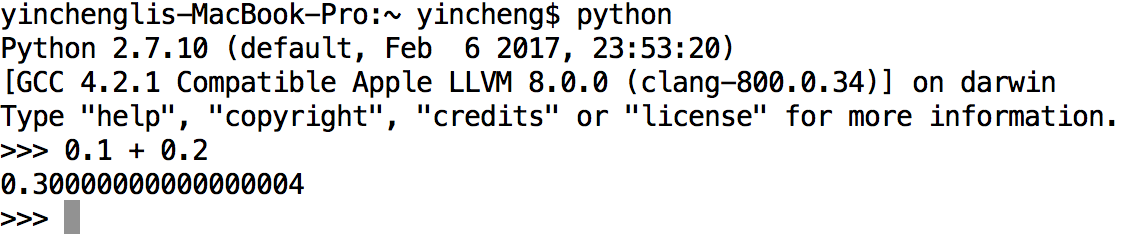0.300000000000000044408920985006261616945266723632812500000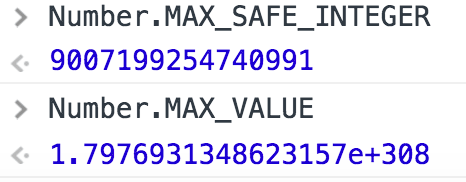JS能表示的最大整数为9e16，能表示的最大正数为1.79e308，这两个数是怎么得来的呢？先来看一下整数在计算机的存储方式。

32位有符号整型的存储方式如下图所示：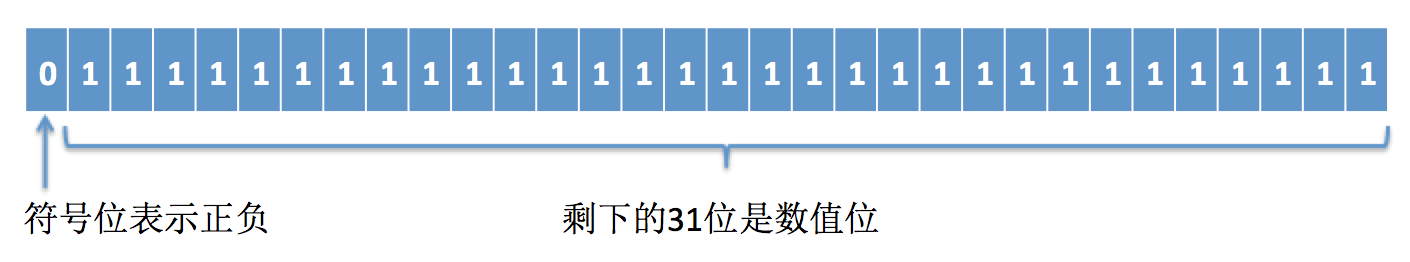2 ^ 31 – 1 = 2147483647

2 ^ 63  – 1 = 9223372036854775807

0.75 = 1.1 * 2 ^ -1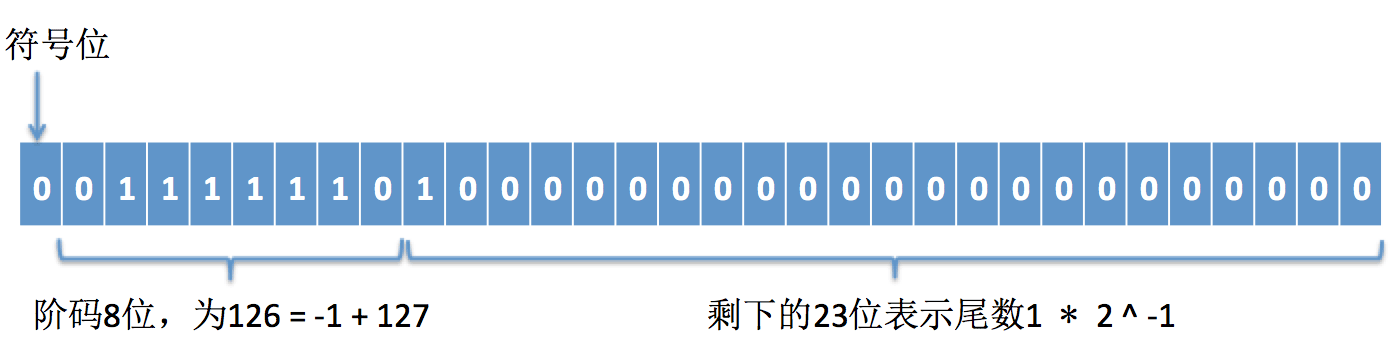(1 + 1 * 2 ^ – 1) * 2 ^ (126 – 127) = 1.5 * 2 ^ -1 = 0.75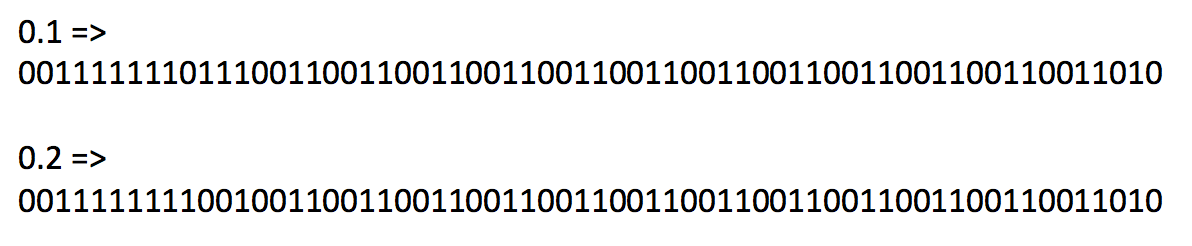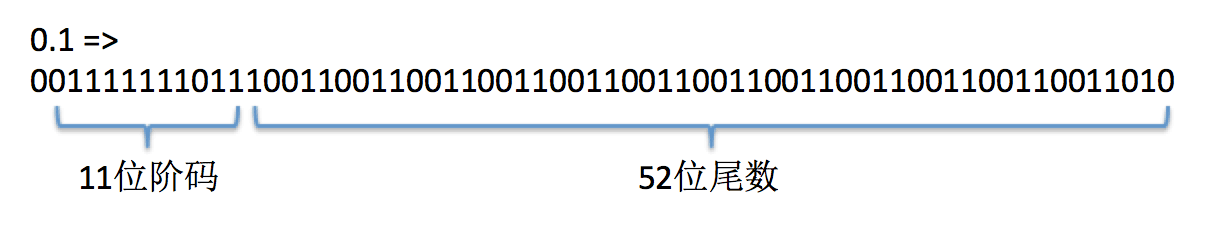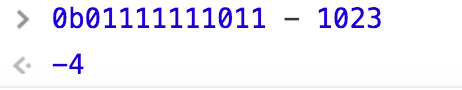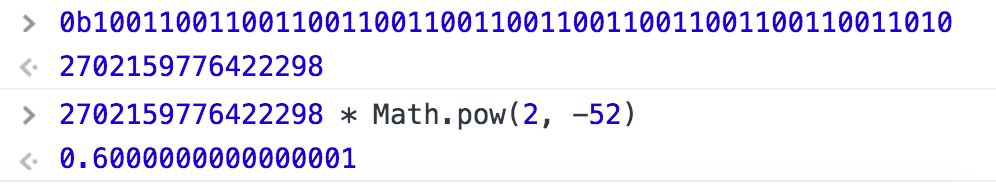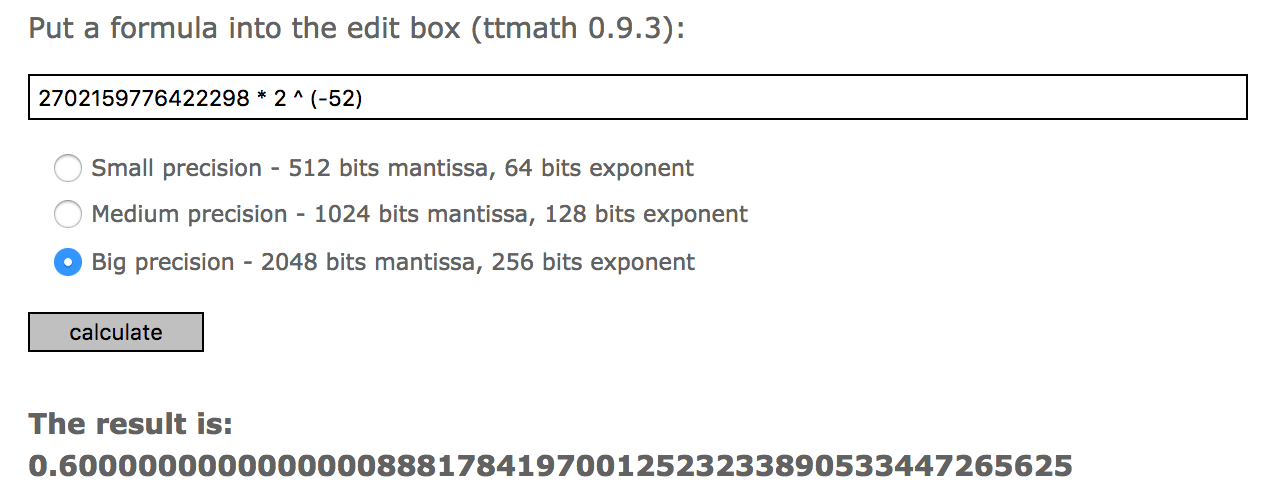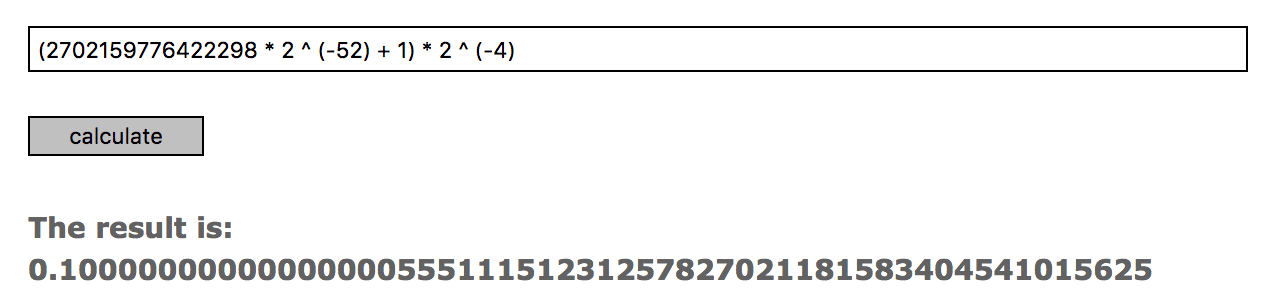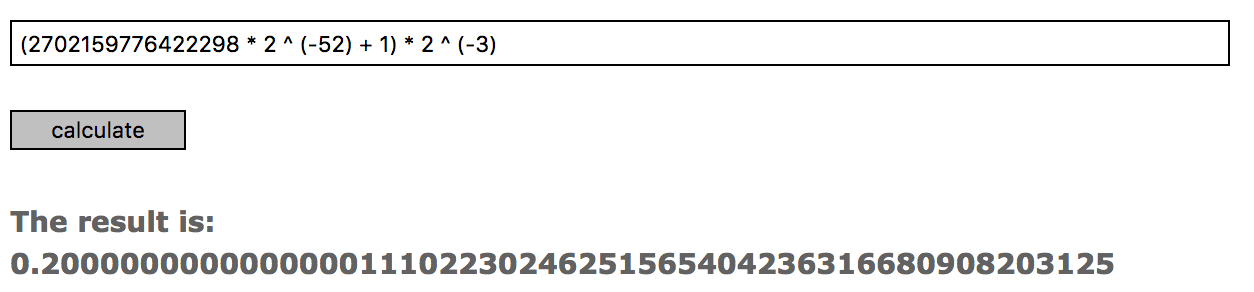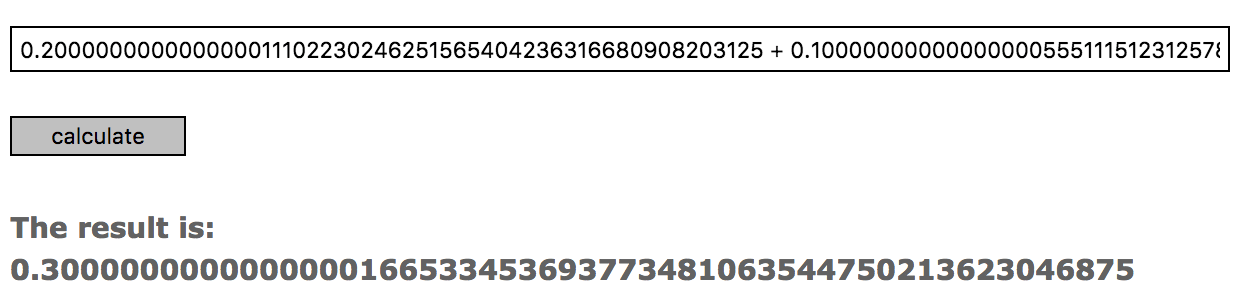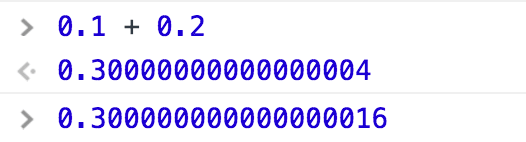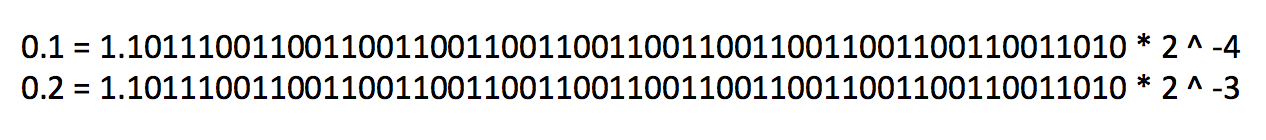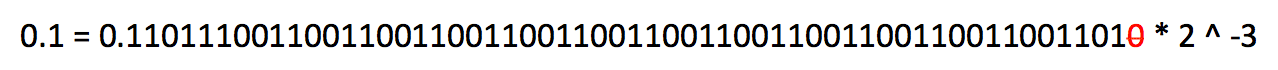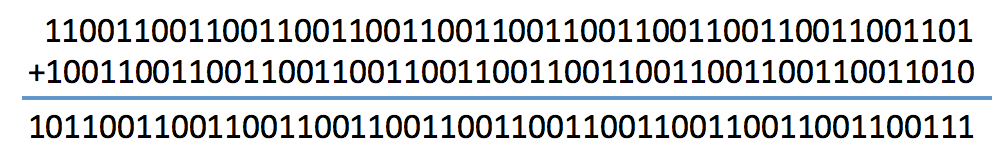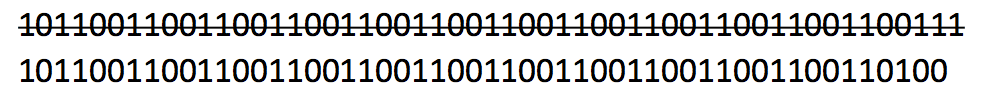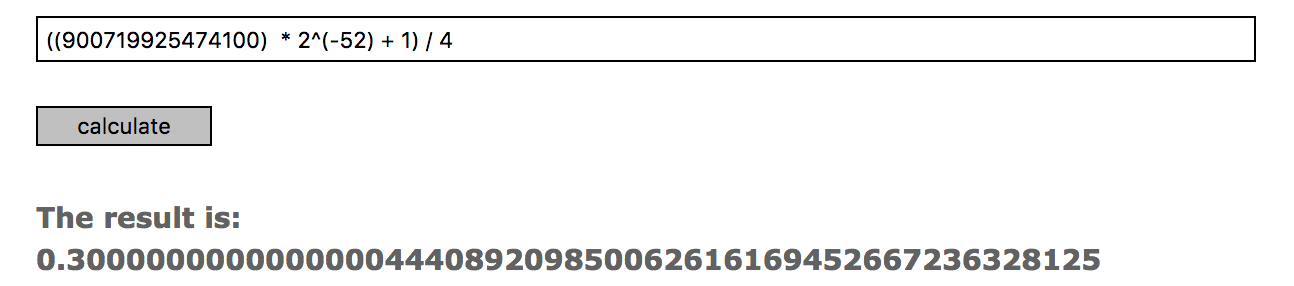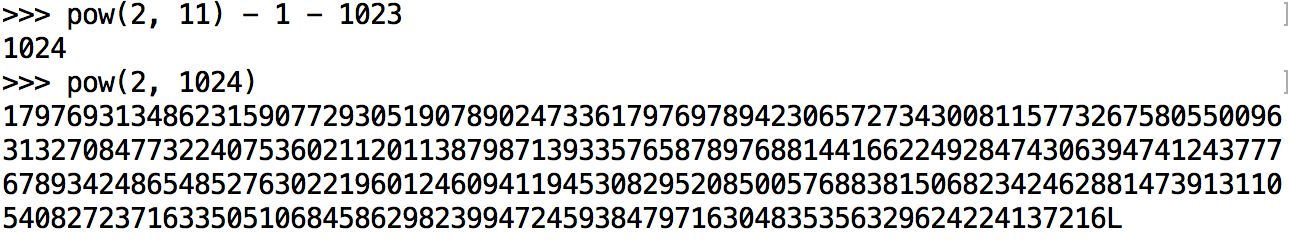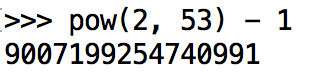最后怎么判断两个小数是否相等呢？用等号肯定是不行的了，判断两个小数是否相等要用它们的差值和一个很小的小数进行比较，如果小于这个小数，则认为两者相等，ES6新增了一个Math.EPSILON属性，如比较0.1 + 0.2是否等0.3应该这么操作：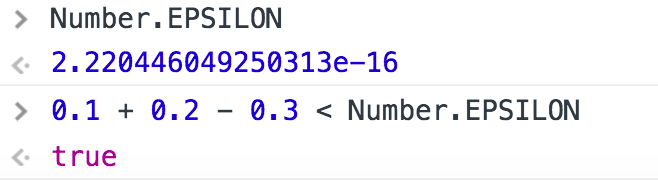### 12 回复 ›

1.parseFloat(1000.555 + ‘1’).toFixed(2)

2.为什么(900719925474100*2^(-52)+1)*2^(-2)这里面要加一呢

3.小白我看的受益匪浅，有两个地方是书写失误了吗？
“0.75 = 1.1 * 2 ^ -1 ” 这个地方 是不是 “0.75 = 1.5 * 2 ^ -1 ”，

“0.1的阶码为01111111011，等于二进制1019” 应该是“。。。。。，等于十进制1019”

•0.75 = 1.1 * 2 ^ -1这个应该是对的，因为我说的是二进制
第二个你说得对，写错了，应该是十进制

•你好，感谢您写的文章，对我的帮助很大。但是好像有有两个图不对，还请不吝赐教。
如上面的0.1是小阶，需要对它进行处理，如下：

这个图好像不对，是否应该是
0.1=1.1001100110011001100110011001100110011001100110011010*2^(-4)
0.2=1.1001100110011001100110011001100110011001100110011010*2^(-3)
需要把0.1的小数点向左移一位变成：

0.1=0.11001100110011001100110011001100110011001100110011010*2^(-3)

再次感谢您的付出。

•你好，感谢您写的文章，对我的帮助很大。但是好像有两个图不对，还请不吝赐教。
如上面的0.1是小阶，需要对它进行处理，如下：

这个图好像不对，是否应该是
0.1=1.1001100110011001100110011001100110011001100110011010*2^(-4)
0.2=1.1001100110011001100110011001100110011001100110011010*2^(-3)
需要把0.1的小数点向左移一位变成：

0.1=0.11001100110011001100110011001100110011001100110011010*2^(-3)

再次感谢您的付出。